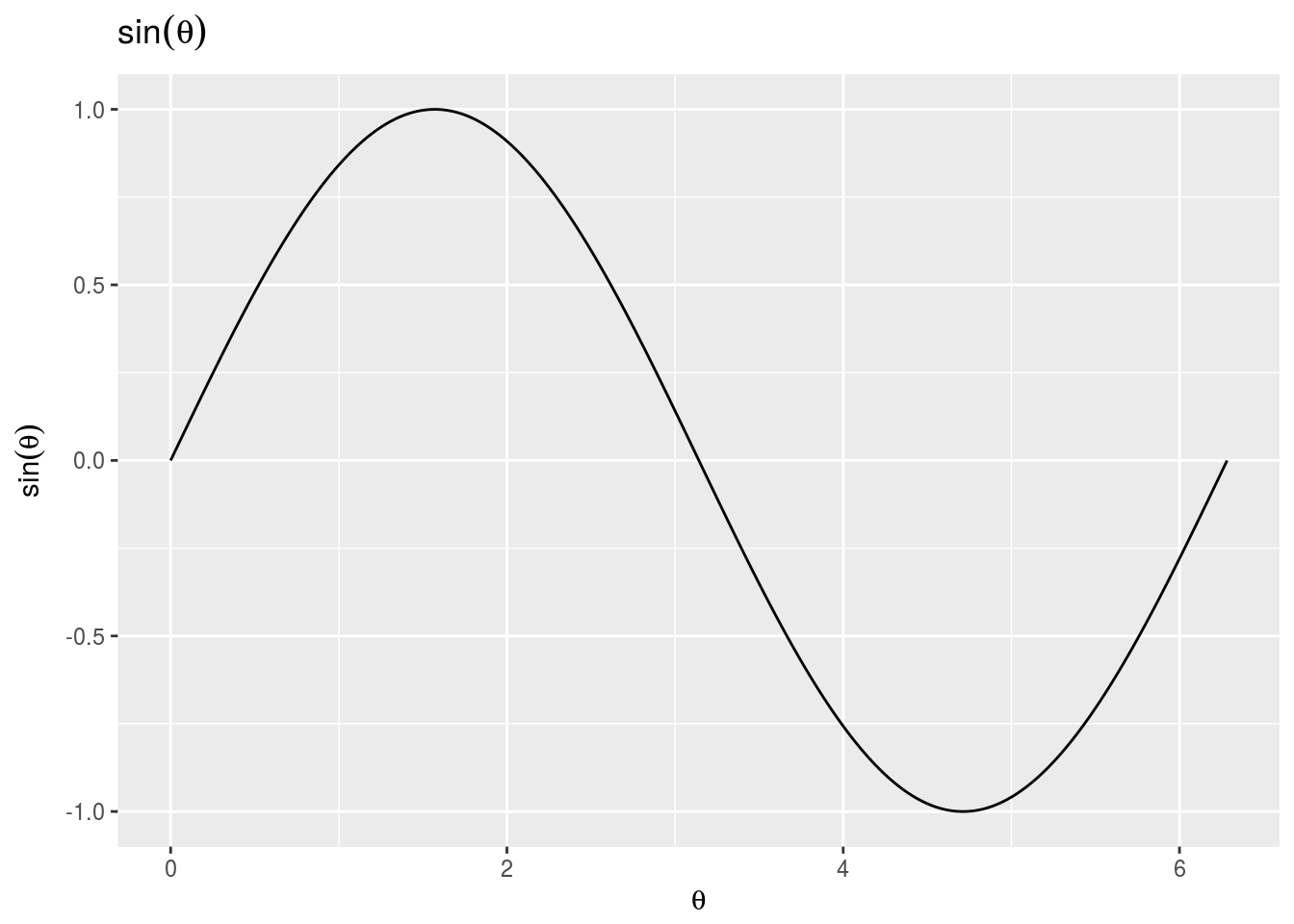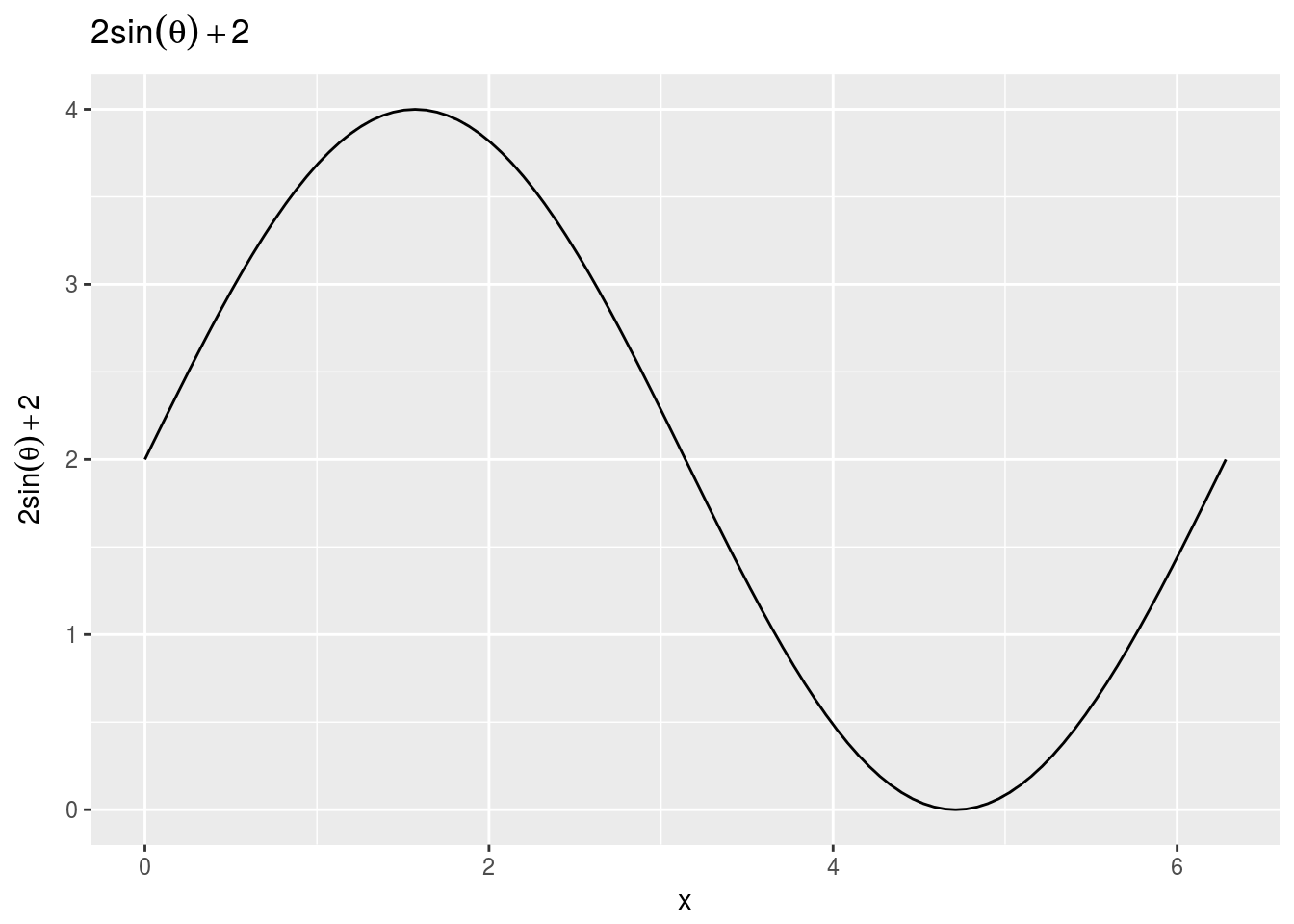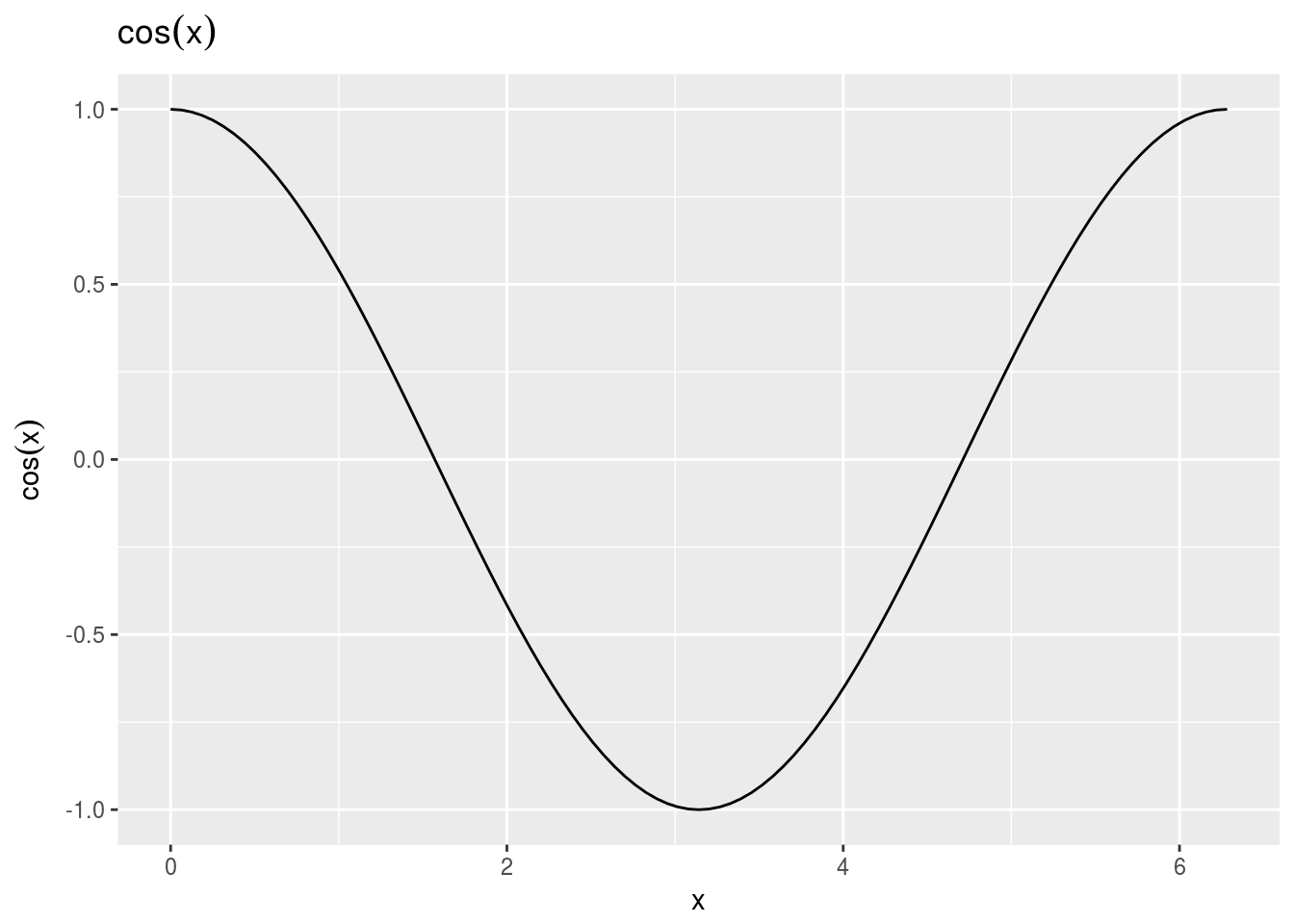Note: If you wish to replicate the R code below, then you will need to copy and paste the following commands in R first (to make sure you have all the packages and their dependencies):

``````install.packages("ggplot2")
# install the ggplot2 package

library(ggplot2)``````

This document was created with rmarkdown 1.2 using the following:

• R 3.3.2 (2016-10-31)
• ggplot2 2.2.0

### 1) theta = 0:2*pi; plot(theta, sin(theta)) in GNU Octave/MATLAB (Brockman 443)

``````library(ggplot2)

# in R using the ggplot2 plotting function
theta <- seq(0, 2, by = 0.001) * pi

p <- qplot(theta, sin(theta), geom = "line", main = expression(sin(theta))) +
xlab(expression(theta))
p <- p + ylab(expression(sin(theta)))
print(p)``````### 2) fplot(’2*sin(theta)+2’, [0 2*pi]) in GNU Octave/MATLAB (Brockman 443)

``````library(ggplot2)

# in R using the ggplot2 plotting function
f1 <- function(theta) 2 * sin(theta) + 2

ggplot(data.frame(x = c(0, 2 * pi)), aes(x)) + stat_function(fun = f1) + labs(x = "x",
y = expression(2 * sin(theta) + 2), title = expression(2 * sin(theta) +
2))``````### 3) fplot(@cos, [0, 2*pi]) in GNU Octave/MATLAB (Eaton 258)

``````library(ggplot2)

# in R using the ggplot2 plotting function
ggplot(data.frame(x = c(0, 2 * pi)), aes(x)) + stat_function(fun = cos) + labs(x = "x",
y = expression(cos(x)), title = expression(cos(x)))``````### 4) fplot(“[cos(x), sin(x)]”, [0 2*pi]) in GNU Octave/MATLAB (Eaton 258)

``````library(ggplot2)

# in R using the ggplot2 plotting function

ggplot(data.frame(x = c(0, 2 * pi)), aes(x)) + stat_function(fun = cos, aes(colour = "cos(x)")) +
stat_function(fun = sin, aes(colour = "sin(x)")) + labs(x = "x", y = "y") +
scale_colour_manual("Legend", values = c("red", "blue"), labels = c(expression(cos(x),
sin(x))))``````